# RGB_LED_Module__SKU_DFR0124_-DFRobot

## introduction

This Arduino-compatible module uses three TI TLC5940 PWM LED chips to drive a 4x4 array of RGB LEDs. Each color is controlled by a different chip so you can adjust the reference resistors for the appropriate current. The TLC5940 chips are not directly connected to any Arduino pins, but a header is provided for you to make a manual connection depending on the needs of your sketch.

The module can be serially linked to make a wall or circle. (Up to 16 modules)

This module comes with assemblied and can be directly plugged into our Interface shield. The link is conducted by IDC2x5 cables. It can also be directly connected to Arduino via the F/M cables.

## Specifications

• 16 Channels
• 12 bit (4096 Steps) Grayscale PWM Control
• Dot Correction
• 6 bit (64 Steps)
• Storable in Integrated EEPROM
• Drive Capability (Constant-Current Sink)
• 0 mA to 60 mA (VCC < 3.6 V)
• 0 mA to 120 mA (VCC > 3.6 V)
• LED Power Supply Voltage up to 17 V
• VCC = 3 V to 5.5 V
• Serial Data Interface
• Controlled In-Rush Current
• 30MHz Data Transfer Rate
• CMOS Level I/O
• Error Information
• LOD: LED Open Detection The LED open detection
• TEF: Thermal Error Flag

## Connection diagram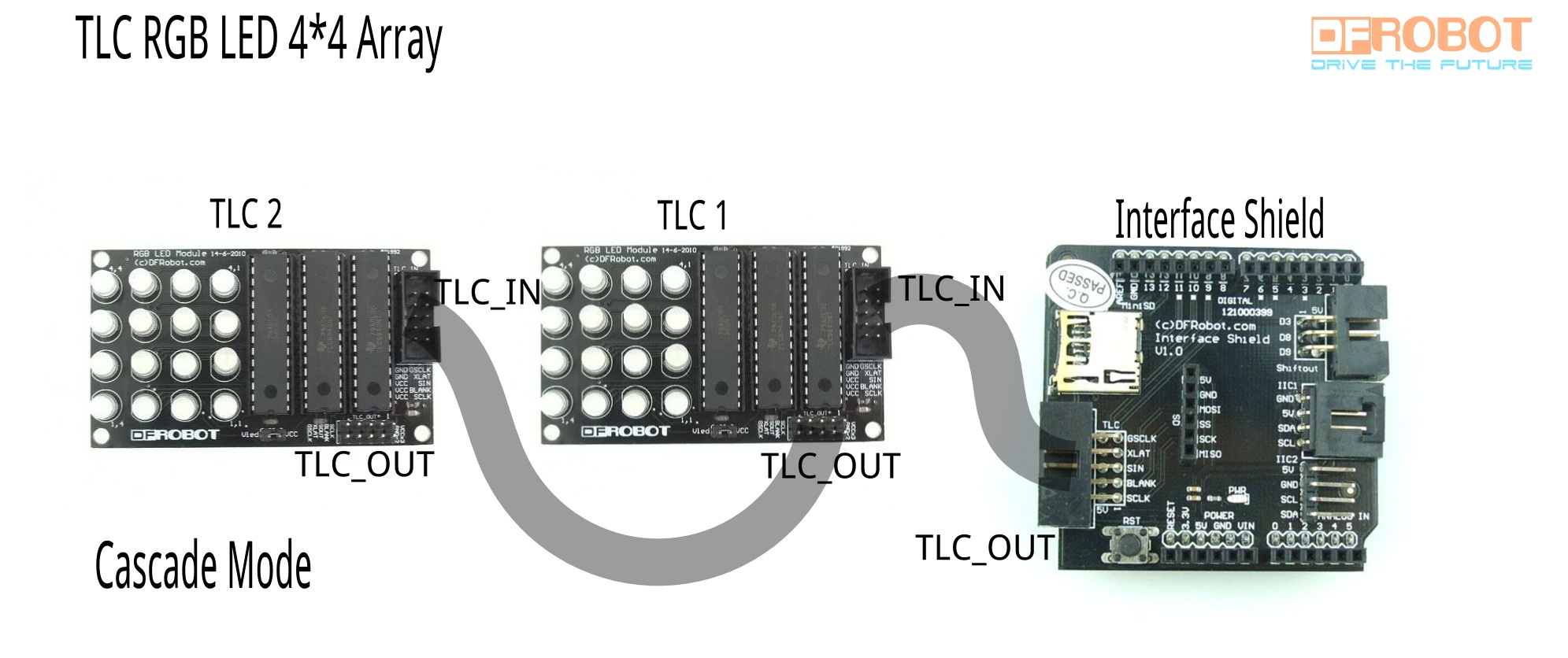## User guides

### Hardware preparation

If you have interface shield, just plug in IDC2x5 cables. Please see connection diagram.

Otherwise see Basic Pin setup:

ARDUINO ARDUINO u u u u
13 -> SCLK (pin 25) OUT1 1 28 OUT channel 0
12 OUT2 2 27 -> GND (VPRG)
11 -> SIN (pin 26) OUT3 3 26 -> SIN (pin 11)
10 -> BLANK (pin 23) OUT4 4 25 -> SCLK (pin 13)
9 -> XLAT (pin 24) . 5 24 -> XLAT (pin 9)
8 . 6 23 -> BLANK (pin 10)
7 . 7 22 -> GND
6 . 8 21 -> VCC (+5V)
5 . 9 20 -> 2K Resistor -> GND
4 . 10 19 -> +5V (DCPRG)
3 -> GSCLK (pin 18) . 11 18 -> GSCLK (pin 3)
2 . 12 17 -> SOUT
1 . 13 16 -> XERR
0 OUT14 14 15 OUT channel 15

If you are daisy-chaining more than one TLC, connect the SOUT of the first TLC to the SIN of the next.All the other pins should just be connected together:

`BLANK on Arduino -> BLANK of TLC1 -> BLANK of TLC2 -> ...`

`XLAT on Arduino -> XLAT of TLC1 -> XLAT of TLC2 -> ...`

• The one exception is that each TLC needs it's own resistor between pin 20 and GND.This library uses the PWM output ability of digital pins 3, 9, 10, and 11. Do not use analogWrite(...) on these pins.

### Software preparation

• Download the Arduino library. It includes several head files. The most fundamental ones are "Tlc5940.h" and "tlc_config.h". The first one contains the most basic functions to control the LED arrays and the second one is variable declaration.

## Sample code

• Notation:
• Change the value of defined invariable NUM_TLCS ( In the file: "tlc_config.h" incuded in the library folder: "Tlc5940".) from 3 to 6(if you want cascade two LED modules), to 9(if you want cascade three LED modules)...
• The order of channel is determined by the order of TLC microcontroller. channnel 0-15 are 16 red LEDs controlled by the first TLC microcontroller. channel 16-31 are 16 green LEDs, channel 32-47 are 16 blue LEDs. One RGB modules has 3 TLC microcontrollers and if you want to use two RGB LED modules, channel 48-63 are 16 red LEDS of second module, and so on. See function: Tlc.set(channel,brightness). This is the function which turns one specific LED on.
• Let's see sample 1.

Sample 1 is a demo of controllable LED color tuning and fading. It uses the library "tlc_fades.h". If you have a potentiometer,it will be better. Otherwise, you can connect the analog port 0 to 5V or ground to observe the changes. Substitute the parameter "TLC_FADE_BUFFER_LENGTH" in head file "tlc_fades.h" to 40.

``````/*
Description: Fades a line down the channels, with max value and duration based on
the voltage of analog pin 0.
See the BasicUse example for hardware setup.
This test demo is  bases on  the example "fades" and it should include head file "tlc_fades.h". ( Written by Alex Leone <acleone ~AT~ gmail.com>, 2009-02-03 )
Here there's something else we can improve:
1. Connect a potentiometer to the analog 0 is better. We can see what will happen if we tune the rotary switch.
2. Change the value of defined invariable NUM_TLCS  ( In the file: "tlc_config.h" incuded in the library folder: "Tlc5940".) from 3 to 6(if you want cascade two LED modules), to 9(if you want cascade three LED modules)...
3. The order of channel is determined by the order of TLC microcontroller. channnel 0-15 are 16 red LEDs controlled by the first TLC microcontroller. channel 16-31 are 16 green LEDs, channel 32-47 are 16 blue LEDs.
One RGB modules has 3 TLC microcontrollers and if you want to use two RGB LED modules, channel 48-63 are 16 red LEDS of second module, and so on.
4. "tlc_fades.h" is well designed, easy to use but there's not much room for us to change the programming style arbitrarily. The most interesting point in this code is no delaying at all. It use the slight loop calculating
time of computer and  returning function millis() to achieve the effect of delay. And the most important character in head file "tlc_fades.h" is "TLC_FADE_BUFFER_LENGTH" (The length of struct array: tlc_fadeBuffer[]).
We can change the length a little bit larger. For example, change it from 24 to 40! The fading will be even smoother if we turn the analog 0 to the max value.You can try if by changing this length. However, there's one
notation: the length cannot be extremely large because struct array is very space consuming! In arduino, global variable is stored in SRAM and SRAM is usually 2Ks or 3Ks.    .
Author: Michael from DFrobot <zhyp90211@gmail.com>, 2012-02-06
*/
//#####################include##########################################################################
#include "Tlc5940.h"
//######################################################################################################
TLC_CHANNEL_TYPE channel;

void setup()
{
Tlc.init();
}

void loop()
{
if (!tlc_isFading(channel)) {  //update if and only if the fading of this channel is done or hasn't started.
uint16_t duration = analogRead(0) * 2;     // the duration of fading is determined by potentiometer.
int maxValue = analogRead(0) * 1.5;        // the brightness of fading is determined by potentiometer.
uint32_t startMillis = millis() + 50;
uint32_t endMillis = startMillis + duration;
if(channel<16)
{maxValue=maxValue*1.5;} //here we make red light brighter.
if(channel>=16&&channel<32)
{maxValue=maxValue*0.4;} //here we make green light darker.The purpose of it is just making the light fading look more comfortable.
}
if (channel++ == NUM_TLCS * 16) {  //It keeps searching the struct array: tlc_fadeBuffer[NUM_TLCS * 16] defined in "tlc_fades.h" begging from channel 0.
channel = 0;
}
}
delay(100);
tlc_updateFades(); //This is what LEDs are actually lighted.
}``````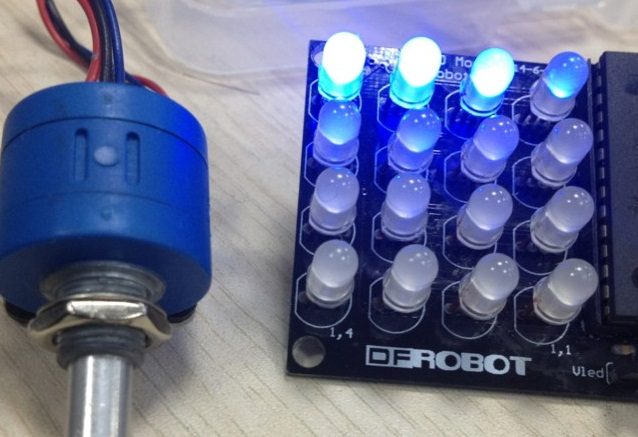• Let's see sample 2. Sample 2 needs another two headfiles "basicProcess.h" and "message.h". Copy them under the same folder of the Arduino code. It demonstrates simple pattern animation, rolling, etc. Notation, here we need two LED modules.
• Arduino code
``````/*
Description:    This is a demo of using 4*4 RGB LED written by Arduino 1.0. First, you will see a pattern of  face. Then, eyes are blinking. After that a red heart appears. Finally, you will see a rolling word: love.
Guide:          1. You'd better read the example "basic use" in this library before trying to modify the code as your version.
2. Put head files: basicProcess.h and message.h under the same folder of LEDArrayTEST2.
3. The order of channel is determined by the order of TLC microcontroller. channnel 0-15 are 16 red LEDs controlled by the first TLC microcontroller. channel 16-31 are 16 green LEDs, channel 32-47 are
16 blue LEDs. In our project, one RGB modules has 3 TLC microcontrollers and we use two RGB LED modules with 6 TLCS totally.Thus, channel 48-63 are 16 red LEDS of second module, and so on.
4. Change the value of defined invariable NUM_TLCS from 3 to 6( In the file: "tlc_config.h" incuded in the library folder: "Tlc5940".) since we need two modules for this demo.
5. "basicProcess.h" is mapping the channel into a more friendly structure so that you can use x,y axis location to process the data.
6. "message.h"  includes the message of word: love. You can change the word to whatever you want(any word, pattern, shape) by creating x,y axis location information first.
Author:         Michael from DFrobot
Contact e-mail: zhyp90211@gamil.com
Date:           2012/02/08
*/
//##########include##########################################################################################################
#include "Tlc5940.h"
#include "basicProcess.h"
#include "message.h"

//########function declaration##########################################################################################################
void face(CHANNEL *ptr,int unit, int brightness, COLOR color); //face pattern (channel structureis defined in "basicProcess.h", unit is the shifting amount)
void heart(CHANNEL *ptr,COLOR color,int brightness);  //heart pattern

//##########setup##################################################################################################################
void setup()
{
/* Call Tlc.init() to setup the tlc.
You can optionally pass an initial PWM value (0 - 4095) for all channels.*/
Tlc.init();
for (int channel=0;channel<NUM_TLCS*16;channel++)
{
mapping(channel);

}
//###########face####################################################################################################
CHANNEL* ptr;
for(int i=-1;i<10;i++) // -1 is the starting location and 10 is the ending location of moving face, you can change it to see what happens
{
for (ptr=array;ptr<array+NUM_TLCS*16;ptr++)
{
face(ptr,i,BLUE,1000,false);     //show moving face
}
Tlc.update();
/* Tlc.update() sends the data to the TLCs.  This is when the LEDs will
actually change. */
delay(200);
Tlc.clear();
}

for(int i=0;i<5;i++)   //5 is the time of eyes'blinking, change it to see the effects
{
for (ptr=array;ptr<array+NUM_TLCS*16;ptr++)
{
face(ptr,1,BLUE,1000,i%2);
}
Tlc.update();
delay(1000);
Tlc.clear();
}

//############heart####################################################################################################
for (ptr=array;ptr<array+NUM_TLCS*16;ptr++)
{
//heart(ptr,GREEN,100);
heart(ptr,RED,3500);

}
Tlc.update();
delay(2000);
Tlc.clear();

MessageInitialize();  //"defined in message.h"

}

//#############loop: word rolling ######################################################################################
void loop()
{

CHANNEL* ptr;
for(int i=-15;i<9;i++)  //starting and ending position of word
{
for (ptr=array;ptr<array+NUM_TLCS*16;ptr++)
{
//heart(ptr,GREEN,100);
loveMessage(ptr,i,200,BLUE);
loveMessage(ptr,i,4000,RED);  //we blend red and blue to make purple, change the color whatever you want
}
Tlc.update();
delay(200);
Tlc.clear();
}
}
//#################################################################################################################
void face(CHANNEL *ptr,int unit,  COLOR color, int brightness, boolean blinking)
{

if(ptr->X==1 && (ptr->Y-1+unit)%8+1==7 && ptr->color==color)
Tlc.set(ptr->channel,brightness);
if(ptr->X==1 && (ptr->Y-1+unit)%8+1==6 && ptr->color==color)
Tlc.set(ptr->channel,brightness);
if(ptr->X==1 && (ptr->Y-1+unit)%8+1==5 && ptr->color==color)
Tlc.set(ptr->channel,brightness);
if(ptr->X==2 && (ptr->Y-1+unit)%8+1==8 && ptr->color==color)
Tlc.set(ptr->channel,brightness);
if(ptr->X==2 && (ptr->Y-1+unit)%8+1==4 && ptr->color==color)
Tlc.set(ptr->channel,brightness);
if(ptr->X==3 && (ptr->Y-1+unit)%8+1==8 && ptr->color==color)
Tlc.set(ptr->channel,brightness);
if(ptr->X==3 && (ptr->Y-1+unit)%8+1==7 && ptr->color==color) //locaton of left eye
{
}
if(ptr->X==3 && (ptr->Y-1+unit)%8+1==5 && ptr->color==color)//location of right eye
{
}
if(ptr->X==3 && (ptr->Y-1+unit)%8+1==4 && ptr->color==color)
Tlc.set(ptr->channel,brightness);
if(ptr->X==4 && (ptr->Y-1+unit)%8+1==7 && ptr->color==color)
Tlc.set(ptr->channel,brightness);
if(ptr->X==4 && (ptr->Y-1+unit)%8+1==6 && ptr->color==color)
Tlc.set(ptr->channel,brightness);
if(ptr->X==4 && (ptr->Y-1+unit)%8+1==5 && ptr->color==color)
Tlc.set(ptr->channel,brightness);
}
//###################################################################################################################

void heart(CHANNEL *ptr,COLOR color,int brightness)
{
if(ptr->X==4 && ptr->Y==6 && ptr->color==color)
Tlc.set(ptr->channel,brightness);
if(ptr->X==4 && ptr->Y==4 && ptr->color==color)
Tlc.set(ptr->channel,brightness);
if(ptr->X==3 && ptr->Y==7 && ptr->color==color)
Tlc.set(ptr->channel,brightness);
if(ptr->X==3 && ptr->Y==6 && ptr->color==color)
Tlc.set(ptr->channel,brightness);
if(ptr->X==3 && ptr->Y==5 && ptr->color==color)
Tlc.set(ptr->channel,brightness);
if(ptr->X==3 && ptr->Y==4 && ptr->color==color)
Tlc.set(ptr->channel,brightness);
if(ptr->X==3 && ptr->Y==3 && ptr->color==color)
Tlc.set(ptr->channel,brightness);
if(ptr->X==2 && ptr->Y==6 && ptr->color==color)
Tlc.set(ptr->channel,brightness);
if(ptr->X==2 && ptr->Y==5 && ptr->color==color)
Tlc.set(ptr->channel,brightness);
if(ptr->X==2 && ptr->Y==4 && ptr->color==color)
Tlc.set(ptr->channel,brightness);
if(ptr->X==1 && ptr->Y==5 && ptr->color==color)
Tlc.set(ptr->channel,brightness);

}

``````
• "basicProcess.h"
``````/*
This headfile transfer channel into two dimensional x,y axis location and corresponding color.
the x,y axis information is as follows:
(4,4) (4,3) (4,2) (4,1)
(3,4) (3,3) (3,2) (3,1)
(2,4) (2,3) (2,2) (2,1)
(1,4) (1,3) (1,2) (1,1)
if we have more than one module:
...(4,9)    (4,8) (4,7) (4,6) (4,5)    (4,4) (4,3) (4,2) (4,1)
...(3,9)    (3,8) (3,7) (3,6) (3,5)    (3,4) (3,3) (3,2) (3,1)
...(2,9)    (2,8) (2,7) (2,6) (2,5)    (2,4) (2,3) (2,2) (2,1)
...(1,9)    (1,8) (1,7) (1,6) (1,5)    (1,4) (1,3) (1,2) (1,1)
*/

#include "Tlc5940.h"

enum COLOR{RED,GREEN,BLUE};
struct CHANNEL
{
int X;
int Y;
int channel;  //(0 - NUM_TLCS*16)
COLOR color;

};

CHANNEL array[NUM_TLCS*16];

void mapping(int channel)
{

CHANNEL* value=array+channel;
//red
if((channel%48)<16)   //48 is used to determine whether one module or multi modules. ( One module has 3 colors and each color has 16 LEDs.)
{
value->Y=(channel%48)%4+channel/48*4+1;
value->X=(channel%48)/4+1;
value->color=RED;
value->channel=channel;
}
//green
if((channel%48)>=16&&(channel%48)<32)
{
value->Y=((channel%48)-16)%4+channel/48*4+1;
value->X=((channel%48)-16)/4+1;
value->color=GREEN;
value->channel=channel;

}
//blue
if((channel%48)>=32&&(channel%48)<48)
{
value->Y=((channel%48)-16*2)%4+channel/48*4+1;
value->X=((channel%48)-16*2)/4+1;
value->color=BLUE;
value->channel=channel;

}

}

``````
• "message.h"
``````
/*
This headfile is for message inputing. Here it is a word: love. Message is initialized using two dimensional array.
for example:
0 1 1 0
1 1 1 1
1 1 1 1
0 1 1 0  can be represented as a circle.
*/
int Message; //the dimension is according to the message you want to input
void MessageInitialize()
{
for(int i=0;i<4;i++)
{
for(int j=0;j<15;j++)
{
Message[i][j]=0;
}
}
Message=1;
Message=1;
Message=1;
Message=1;
Message=1;
Message=1;
Message=1;
Message=1;
Message=1;
Message=1;
Message=1;
Message=1;
Message=1;
Message=1;
Message=1;
Message=1;
Message=1;
Message=1;
Message=1;
Message=1;
Message=1;
Message=1;
Message=1;
Message=1;
Message=1;
}

void loveMessage(CHANNEL *ptr,int unit,int brightness, COLOR color)  //"unit" is for horizontal shifting
{
for(int i=0;i<4;i++)
{
for(int j=0;j<15;j++)
{
if(Message[i][j]==1)
{

if(ptr->X==i+1 && ptr->Y==j+1+unit && ptr->color==color)
Tlc.set(ptr->channel,brightness);
}
}
}
}

``````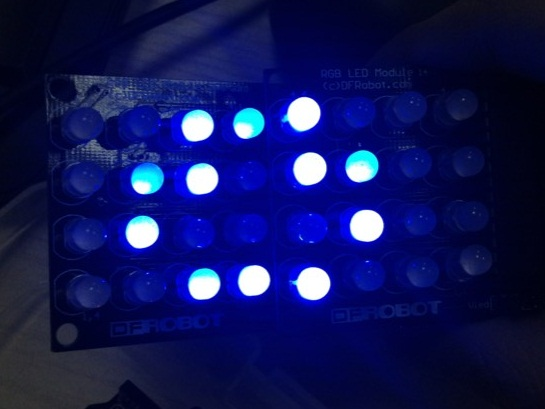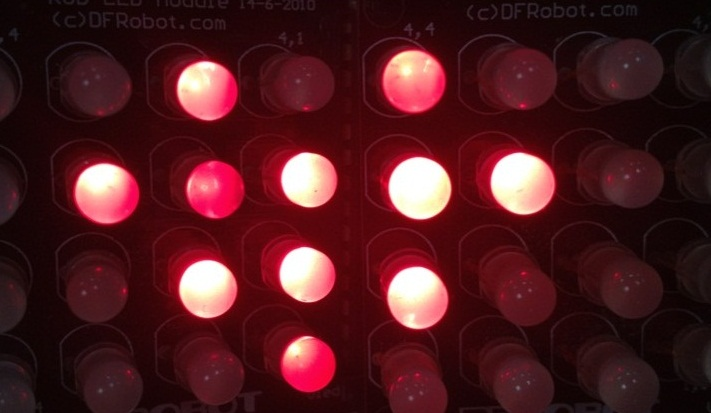## Documentation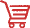Get RGB LED Module from DFRobot Store or DFRobot Distributor.